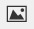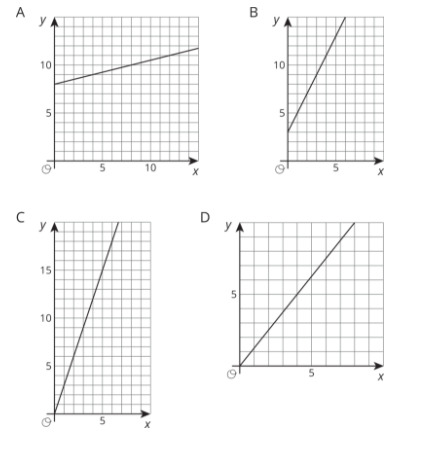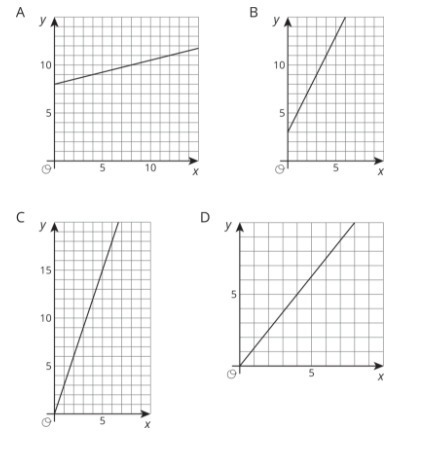PSABM5VZ - Practice---Acc7.5 Lesson 7 Translating to y = mx + b (8.EE.B, 8.G.A.1)

Select all equations that have graphs with the same y-intercept.

Select all that apply:
Part A)

Create a graph showing the equationsandDraw your graph on paper, take a picture, and upload it using the image upload iconIf you do not have the ability to upload an image of your work type "Graph is on paper."

Part B)

Explain how the graphs are the same and how they are different.

Part A)

A cable company charges $70 per month for cable service to existing customers. Find a linear equation representing the relationship between x, the number of months of service, and y, the total amount paid in dollars by an existing customer. Complete the equation below y = _______________ Use x as your variable. copied for free from openupresources.org Type your answer below (mathematical expression): Part B) For new customers, there is an additional one-time$100 service fee. Repeat the previous problem for new customers.

Complete the equation below

y = _______________

Part C)

When the two equations are graphed in the coordinate plane, how are they related to each other geometrically?

Part A)

A mountain road is 5 miles long and gains elevation at a constant rate. After 2 miles, the elevation is 5500 feet above sea level. After 4 miles, the elevation is 6200 feet above sea level.

Find the elevation of the road at the point where the road begins.

Type your answer below as a number (example: 5, 3.1, 4 1/2, or 3/2):
Part B)

Describe where you would see the point in part (a) on a graph where represents the elevation in feet and x represents the distance along the road in miles.

Part A)

Match the given situation to one of the graphs below.

The graph represents the perimeter, y, in units, for an equilateral triangle with side length of x units. The slope of the line is 3.Select one:
Part B)

Match the given situation to one of the graphs below.The amount of money, y, in a cash box after x tickets are purchased for carnival games. The slope of the line is 1/4.

Select one:
Part C)

Match the given situation to one of the graphs below.The number of chapters read, y, after x days. The slope of the line is 5/4.

Select one:
Part D)

Match the given situation to one of the graphs below.The graph shows the cost in dollars, y, of a muffin delivery and the number of muffins, x, ordered. The slope of the line is 2.

Select one: